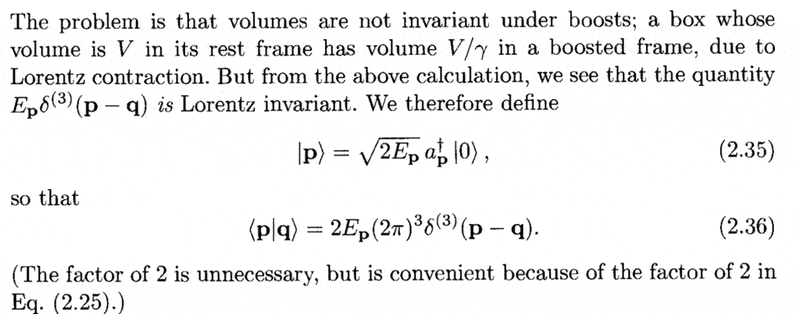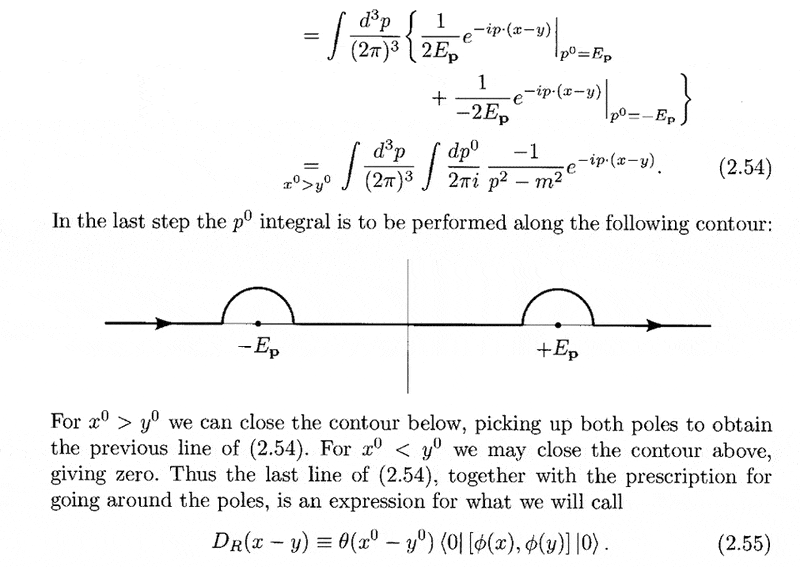# Two stupid questions about Peskin's QFT

Beginner_2010

## Homework Statement

Hi,

I have two stupid questions about Peskin's QFT book.

(1) P23, How to derive from (2.35) to (2.36)
(2) P30, How to derive (2.54)

## Homework Equations

(1)(2)## The Attempt at a Solution

(1) If I consider the dual-space vector, $$\langle \mathbf{q} | = \sqrt{2 E_{\mathbf{q} }} \langle 0 | a_{\mathbf{q}}$$

Combine with the ket (2.35), obtain
$$\langle\mathbf{q} | \mathbf{p} \rangle = 2 \sqrt{ E_{\mathbf{q}} E_{\mathbf{p} } } \langle 0 | a_{\mathbf{q}} a_{\mathbf{p}}^{\dag} | 0 \rangle = 2 \sqrt{ E_{\mathbf{q}} E_{\mathbf{p} } } (2 \pi)^{3} \delta^{(3)} (\mathbf{p} - \mathbf{q})$$

Therefore
$$\langle \mathbf{p} | \mathbf{q} \rangle = \langle \mathbf{q} | \mathbf{p} \rangle^* = 2 \sqrt{ E_{\mathbf{q}} E_{\mathbf{p} } } (2 \pi)^{3} \delta^{(3)} (\mathbf{p} - \mathbf{q})$$

But Peskin's (2.36) has a prefactor $$2 E_{\mathbf{p}}$$ instead of $$2 \sqrt{ E_{\mathbf{q}} E_{\mathbf{p} } }$$, is that made to be the convention?

(2) Is that the principal value of integral $$\int_{- \infty}^{+\infty} d p^0$$ or including the little semi-cycles around $$-E_{\mathbf{p}}$$ and$$+E_{\mathbf{p}}$$ ? If includes the semi-cycles, i can get the result

Thank you ^_^

Staff Emeritus
Homework Helper

## The Attempt at a Solution

(1) If I consider the dual-space vector, $$\langle \mathbf{q} | = \sqrt{2 E_{\mathbf{q} }} \langle 0 | a_{\mathbf{q}}$$

Combine with the ket (2.35), obtain
$$\langle\mathbf{q} | \mathbf{p} \rangle = 2 \sqrt{ E_{\mathbf{q}} E_{\mathbf{p} } } \langle 0 | a_{\mathbf{q}} a_{\mathbf{p}}^{\dag} | 0 \rangle = 2 \sqrt{ E_{\mathbf{q}} E_{\mathbf{p} } } (2 \pi)^{3} \delta^{(3)} (\mathbf{p} - \mathbf{q})$$

Therefore
$$\langle \mathbf{p} | \mathbf{q} \rangle = \langle \mathbf{q} | \mathbf{p} \rangle^* = 2 \sqrt{ E_{\mathbf{q}} E_{\mathbf{p} } } (2 \pi)^{3} \delta^{(3)} (\mathbf{p} - \mathbf{q})$$

But Peskin's (2.36) has a prefactor $$2 E_{\mathbf{p}}$$ instead of $$2 \sqrt{ E_{\mathbf{q}} E_{\mathbf{p} } }$$, is that made to be the convention?
The delta function is non-zero only when p=q, so Ep=Eq.
(2) Is that the principal value of integral $$\int_{- \infty}^{+\infty} d p^0$$ or including the little semi-cycles around $$-E_{\mathbf{p}}$$ and$$+E_{\mathbf{p}}$$? If it includes the semi-cycles, I can get the result.

Beginner_2010
Thank you!